## Appendix ASimilarity Classes of Matrices

The classification of representations of GLn(Fq) is closely analogous to the classification of conjugacy classes. The results in this chapter give a classification of the conjugacy classes in GLn(Fq), along with representatives for each class. Descriptions of the centralisers are also given.

### A.1. Basic properties of matrices

Let F be any field.

Definition A.1. Two matrices A and B with entries in F are said to be similar if there exists an invertible matrix X such that BX = XA.

Similarity is an equivalence relation on the set of all n×n matrices. The equivalence classes are called similarity classes. Given a matrix A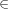Mn(F), for every vector xFn and every polynomial f(t)F[t] define fx = f(A)x. This endows Fn with the structure of an F[t]-module, which will be denoted by MA.

Exercise A.2. If A is similar to B, then MA is isomorphic to MB as an F[t]-module.

Conversely, given an F[t]-module M, pick any basis of M as an F-vector space. Let AM be the matrix by which t acts on M with respect to this basis. A different basis of M would give rise to a matrix similar to AM. Therefore, M determines a similarity class of matrices.

Proposition A.3. AMA gives rise to a bijection between the set of similarity classes of matrices and the set of isomorphism classes of F[t]-modules.

Definition A.4 (Simple matrix). Recall that an F[t]-module is called simple if there is no non-trivial proper subspace of M which is preserved by F[t]. A matrix A is said to be simple if MA is a simple F[t]-module.

Exercise A.5. Show that A is simple if and only if its characteristic polynomial is irreducible.

Exercise A.6. For any two matrices A and B, let AB denote the block matrix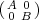. AB will be called the direct sum of A and B. Show that MAB = MA MB (a canonical isomorphism of F[t]-modules).

Definition A.7 (Indecomposable matrix). A matrix is said to be indecomposable if it is not similar to a matrix of the form A B, where A and B are two strictly smaller matrices. Equivalently, A is indecomposable if MA is indecomposable as an F[t]-module.

Definition A.8 (Semisimple matrix). A matrix is said to be semisimple if it is similar to a direct sum of simple matrices. Equivalently, A is semisimple if MA is a semisimple F[t]-module (i.e., MA is a direct sum of simple F[t]-modules).

Exercise A.9. For any λF, show that the matrix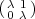is indecomposable, but not semisimple (and hence not simple either).

### A.2. Primary decomposition

Let f(t) be any irreducible monic polynomial in F[t]. Given an F[t]-module M, its f-primary part is the submodule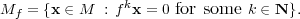Theorem A.10 (Primary decomposition). [Jac84, Theorem 3.11] Let M be an F[t]-module which is also a finite dimensional F-vector space. Then Mf = 0 for all but finitely many irreducible monic polynomials f(t)F[t].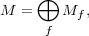the sum being over all the irreducible monic polynomials f for which Mf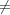0.

Let fF[t] be an irreducible monic polynomial. An F[t]-module M is called f-primary if M = Mf. M is called primary if it is f-primary for some f.

Exercise A.11. Let f(t)F[t] be an irreducible monic polynomial, and p(t)F[t] be any monic polynomial. Show that F[t]∕p(t) is f-primary if and only if p(t) = f(t)r for some r 0.

Theorem A.12. Let f(t)F[t] be an irreducible monic polynomial, and A be a square matrix. Then MfA0 if and only if f(t) divides the characteristic polynomial of A.

Proof. Let χA denote the characteristic polynomial of A. If f is an irreducible polynomial that does not divide χA, then there exist polynomials r and s such that fr+χAs = 1. Evaluating at A and applying the Cayley-Hamilton theorem shows that f(A)r(A) = I. It follows that f(A) is non-singular. Hence f(A)k is also non-singular for every positive integer k. Therefore, MfA = 0.

Conversely, if MfA = 0, then f(A)k is non-singular for every kN. In particular, f(A) is non-singular. Let E be a splitting field of f. Suppose that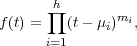with μ1,hE distinct, and m1,,mhN. Therefore,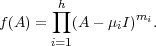Since f(A) is non-singular, so is A - μiI for each i. Therefore, no μi is an eigenvalue of A. It follows that f does not divide χA.

If MA is f-primary then the matrix A is called an f-primary matrix. It follows that a matrix is primary if and only if its characteristic polynomial has a unique irreducible factor.

Corollary A.13. Every matrix AMn(F) is similar to a matrix of the form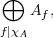where Af is an f-primary matrix, and the sum is over the irreducible factors of the characteristic polynomial of A. Moreover, for every f, the similarity class of Af is uniquely determined by the similarity class of A.

Thus, the study of similarity classes of matrices is reduced to the study of similarity classes of primary matrices.

### A.3. Structure of a primary matrix

Theorem A.14 (Structure theorem). [Jac84, Section 3.8] For every F[t]-module M, there exist non-constant monic polynomials f1,,fr such that f1fr and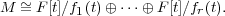Fix an irreducible monic polynomial f(t)F[t]. If M is f-primary, then by Exercise A.11, each for each i, fi = fλi for some λi > 0. Therefore,

Corollary A.15 (Structure of a primary module). If M is an f-primary F[t]-module, then there exists a non-decreasing sequence of integers λ1λr such that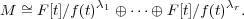Definition A.16 (Partition). A partition is a finite sequence λ = (λ1,r) of positive integers such that λ1λr. Define λ:= λ1 ++ λr. One says that λ is a partition of λ. The length of λ is the non-negative integer r (there is an ‘empty partition’ of length 0 denoted , with ∣∅∣ = 0). Let Λ denote the set of all partitions.

Given a partition λ = (λ1,l), define an F[t]-module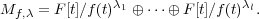Corollary A.15 says that every f-primary F[t]-module is isomorphic to Mf,λ for some partition λ.

Exercise A.17. Suppose that f and fare two irreducible monic polynomials, λ and λtwo partitions. Show that the F[t]-modules Mf,λ and Mf are isomorphic if and only if f = fand λ = λ.

Let S denote the set of all irreducible monic polynomials in F[t]. Given a function ψ : S Λ such that ψ(f) = for all but finitely many fS, let Mφ denote the F[t]-module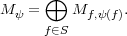Then dimF Mψ = fSdeg(f)ψ(f). Let nψ = dimF Mψ.

Theorem A.18 (Similarity classes of matrices). The map ψMψ is a bijective correspondence between the set of all functions S Λ with the property that ψ(f) = for all but finitely many fS and nψ = n and the set of isomorphism classes of n-dimensional F[t]-modules (and hence the set of similarity classes of n × n matrices).

### A.4. Block Jordan canonical form

There is a version of the Jordan canonical form for matrices for which the irreducible factors of the characteristic polynomial have derivatives which are not identically zero.

In order to obtain this form, we need the following result:

Theorem A.19. Suppose that f an irreducible monic polynomial in F[t] such that f(t) is not identically zero. Let E denote the field F[t]∕f(t). Then the rings k[t]∕f(t)r and E[u]∕ur are isomorphic.

Proof. The main step in the proof is a version of Hensel’s Lemma

Lemma A.20 (Hensel). There exists qr(t)F[t] such that qr(t) t mod f(t), and f(qr(t)) 0 mod f(t)r.

Proof. The proof is by induction on r. When r = 1, one may take q1(t) = t.

Suppose that qr-1(t)F[t] is such that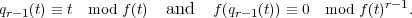Then, using the Taylor expansion, for any h(t)F[t],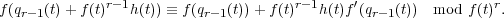Since qr-1(t) t mod f(t), f(qr-1(t) f(t) mod f(t). By hypothesis f(t) is a non-zero polynomial of degree strictly less than f(t). Therefore, f(t) is not divisible by f(t). Since f(t) is irreducible, it means that there exists r(t),s(t)F[t] such that fr + fs = 1, which means that f(t)r(t) 1 mod f(t). Since f(qr-1(t)) 0 mod f(t)r-1, there exists f1(t)F[t] such that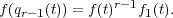When h(t) = -f1(t)r(t) and qr(t) = qr-1(t) + f(t)r-1h(t), one has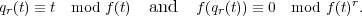Given qr(t) as in Hensel’s lemma, the map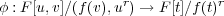given by setting φ(v) = qr(t), and φ(u) = f(t) gives rise to a well defined ring homomorphism, since f(qr(t)) 0 mod f(t)r Since qr(t) t mod f(t), and f(t) and qr(t) lie in the image of φ, t also lies in the image of φ. This makes φ surjective. Moreover, φ is a linear transformation of F-vector spaces of dimension rd. Therefore, φ must be an isomorphism of rings.

Definition A.21 (Companion matrix). Let f(t) = tn-an-1tn-1 --a1t-a0. Then the companion matrix of f is the n × n matrix: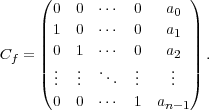Theorem A.22 (Block Jordan Canonical Form). Let AMn(F) be such that for every irreducible factor f of the characteristic polynomial of A, fis not identically zero. Then A can be written as a block diagonal matrix with blocks of the form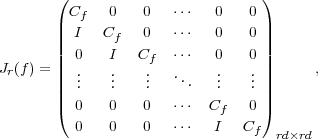where d is the degree of f, an irreducible factor of the characteristic polynomial of A, Cf is the companion matrix of f, and r is a positive integer. Up to rearrangement of blocks, this canonical form is unique.

Proof. By Exercise A.6 and Theorem A.10 one may assume that A is f-primary, for some irreducible monic polynomial f. Let E = F[v]∕f(v). By Corollary A.15 and Theorem A.15, there exists a partition λ such that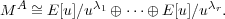In the notation of the proof of Theorem A.19, let θ(t) = t - q(t), where we write q for qλi for some i. Then θ(t)(f(t)). But θ(t)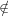(f(t)2), for if it did, we would have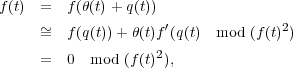a contradiction. Therefore, θ(t) = αf(t), where α is a unit in F[t]∕f(t)λi. In the isomorphism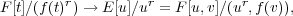tαu + v. Since A acts by t, with respect to the basis of E[u]∕uλi over F given by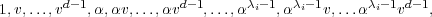the matrix of multiplication by t = αu + v is Jλi(f).

The hypothesis on A in Theorem A.22 always holds when F is a perfect field, as we shall see in Section A.6. By Corollary B.8 every finite field is perfect. Therefore, every matrix over a finite field has a Jordan canonical form.

### A.5. Centralisers

For any AMn(F) define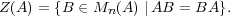Theorem A.23. Let AMn(F) be a matrix such that for each irreducible factor f of the characteristic polynomial of A, fis not identically zero. Suppose that A is similar to fAf, where Af is f-primary (see Corollary A.13). Then Z(A)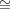fZ(Af). If A is f-primary, E = F[t]∕f(t), and λ is the partition associated to MA in Corollary A.15, then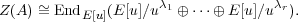Note that the group of units of the centraliser algebra Z(A) will be the centraliser of A in GLn(F).

Proof. The theorem follows easily from Theorem A.19, using the fact that EndF[t]MAZ(A).

### A.6. Perfect fields

Definition A.24. A perfect field is either a field of characteristic zero, or a field of characteristic p > 0 for which the map xxp is bijective.

Theorem A.25. Suppose that F is a perfect field and f(t)F[t] is a non-constant irreducible polynomial. Then f(t) does not vanish identically.

Proof. If f= 0, then the characteristic of F must be p > 0 and f must be of the form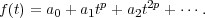Since F is perfect, there exist biE such that bip = ai. Then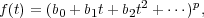contradicting the irreducibility of f.